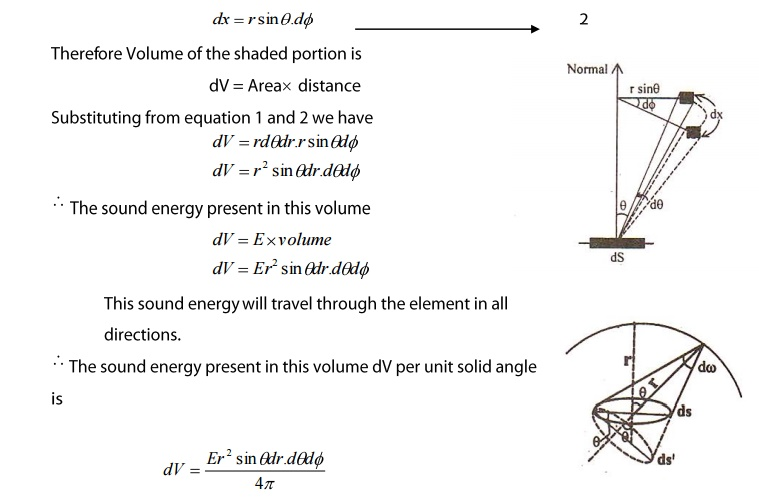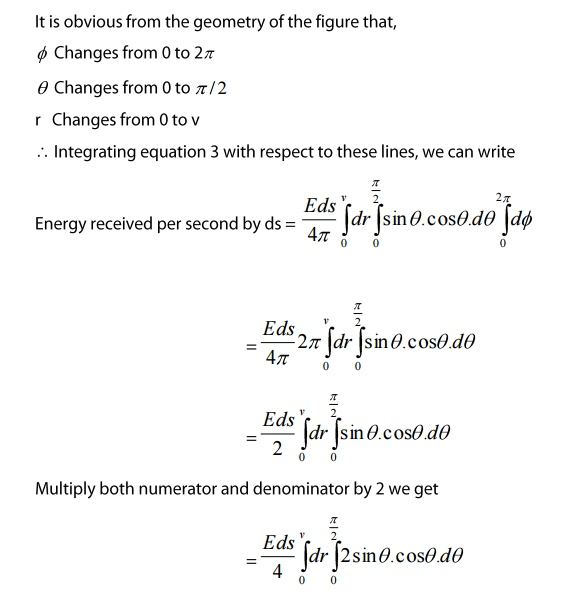Home | | Engineering Physics I | Acoustics off buildings

# Acoustics off buildings

In day today life sound engineering plays a vital role in film industries, broadcasting of television signals and even in television signals. So a new field of science is developedwhich deals with the planning of a building or a hall with a view to provide best audiblesound to the audience and is called Acoustics of building.

Acoustics of buildings

In day today life sound engineering plays a vital role in film industries, broadcasting of television signals and even in television signals. So a new field of science is developedwhich deals with the planning of a building or a hall with a view to provide best audiblesound to the audience and is called Acoustics of building. Therefore to provide a bestaudible sound in a building or hall a prime factor called Reverberation.

1 REVREBERATIONWhen a sound pulse is generated in a hall, the sound wave travels towards all directionand are reflected back by the walls, floors, doors, windows ceiling etc as shown in the figure.

So a sound wave has two to three hundred repeated reflections, before it

becomes inaudible. Therefore, the observer in the hall does not be able to hear a singlesharp sound instead a "role of sound" of diminishing intensity (since part of energy is lostat each reflection)

2 Reverberation time

The duration for which the sound persist is termed as reverberation time

and is measured as the time interval between the sound produced by the source

produced by the source and to the sound wave until it dies.

Definition:

It is defined as the time taken for the sound to fall below the minimum audibility

measured from the instant when the source sound gets stopped.

In designing the auditorium, theatre, conference halls etc, the reverberation time is

the key factor.

If the reverberation time is too large, echoes are produced and if the reverberation

time is too short it becomes inaudible by the observer and the sound is said to be dead.Therefore the reverberation time should not be too large or too short rather it should havean optimum value.

In order to fix this optimum value standard forumla is dervied by W.C.Sabine, who

defined the standard reverberation time as the time taken for the sound to fall to one millionth of its original intensity just before the source is cut off.3 SABINES FORMULA FOR REVERBERRATION

The relation connecting the reverberation time with the volume of the hall, the

area and the absorption coefficient is known a s Sabine's Formula.

Sabine's developed the formula to express the rise and fall of sound intensity by

the following assumptions.

I.            Distribution of sound energy is uniform throughout the hall

II.            There is Interference between the sound waves.

III.            The Absorption coefficient is independent of sound intensity.

IV.            The Rate of emission of sound energy from the source is constant.Let us consider a small element ‘ds’ on a plane wall AB. Assume that the element ds receive the sound energy ‘E’.

Let us draw two concentric circles of radii 'r' and r + dr from the center point 'O' of

ds. Consider a small shaded portion lying in between the two semi circles drawn at an angle θ and θ+dθ, with the normal to ds as shown in the figure.

Let 'dr' be th radial length and rdθ be the arc length

Area of shaded portion rdθdr ---- (1)

If the whole figure is rotated about the normal through an angle ‘dϕ’ as shown in the figure, then it is evident that the area of the shaped portion travels through a small distance dx.To find total energy received by the element ‘ds’ per second, we have to integrate the equation 3 for the whole volume lying within a distance ‘v’ is the Velocity of sound.

It is obvious from the geometry of the figure that,3.1 Growth and Decay of Sound Energy

If ‘P’ is the Power Output (i.e., the rate of emission of sound energy from the source) then we can writeHere Em is the maximum energy from the source (which has been emitted) that is maximum energy which is incident on the wallWhere k is the constant of integration

3.2 Growth of Sound Energy

Let us evaluate for growth

Initially during the growth the boundary conditions

Are at t=0 E=0

Thereore equation 8 becomesWhere Em is the maximum sound energy.

This expression gives the growth of sound energy density ‘E’ with time ‘t’. The growth is along an exponential curve as shown in the figure.3.3 DECAY OF SOUND ENERGY

Let us irst evaluate k or decay.

Here the boundary conditions are at t=0; E=Em

Initially the sound increases from E to Em and now it is going to decay from Em. Therefore time is considered as ‘0’ for E=Em. At E=Emv  the sound energy from the source is cut off. Therefore rate of emission of sound energy from the source=0 i.e., P=0

Therefore from equation 8 we can writeEquation 10 gives the decay of sound energy density with time ‘t’ even after the source is cut off. It is exponentially depressing function from maximum energy(Em) as shown. The growth and decay of sound energy together is represented in the figure.3.4 PROOF OF RREVEREBARTION TIME(T)

According to Sabine, the reverberation time is defined as the time taken by a sound to fall to one millionth of its initial value, when the source of sound is cut off.Equation 13 represents the Reverberation time, which depends on the three factors viz,

i.                   Volume of the hall(V)

ii.                 Surface area(S)

iii.              Absorption coefficient(a) of the materials kept inside the hall.

Among these three actors volume is fixed. Therefore, the reverberation time can be optimized by either varying the surface area of the reflecting surfaces or the absorption coefficient of the materials used inside the hall.

Study Material, Lecturing Notes, Assignment, Reference, Wiki description explanation, brief detail
Physics : Acoustics and Ultrasonics : Acoustics off buildings |